二、空间坐标系及其变换表

 坐 标 系 与 图 形 公 式 与 说 明 [笛卡儿直角坐标系]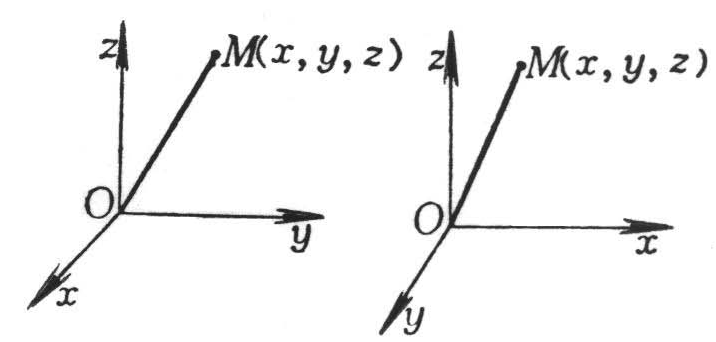右手系 （b）左手系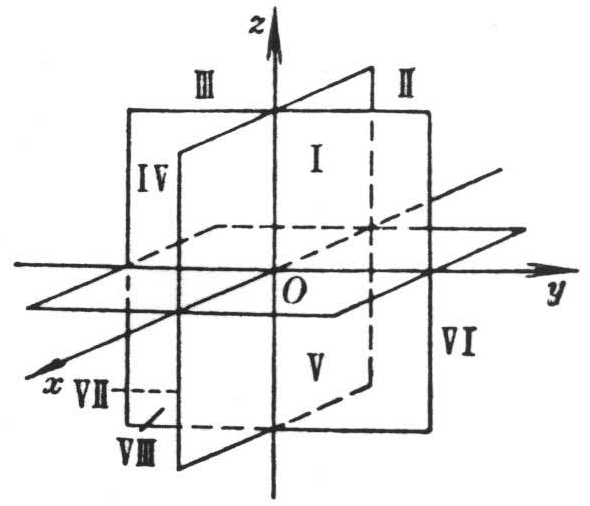(c) Ox为横轴，Oy为纵轴，Oz为竖轴 `M(x, y, z) ` ` ` x为横坐标 y为纵坐标 z为竖坐标 ` ` ` `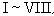为八个卦限，在各个卦限里点的坐标x, y, z的符号为 卦限` x` ` y` ` z ` `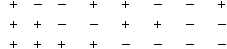` 坐 标 系 与 图 形 公 式 与 说 明 [圆柱面坐标系]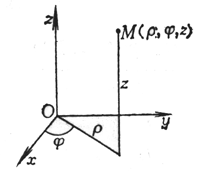r ,j 为点M在Oxy平面上投影的极坐标，z为点M到Oxy平面的距离.这里 0 £ r < ¥ -¥ < j < ¥ -¥ < z < ¥ [球面坐标系(极坐标系)]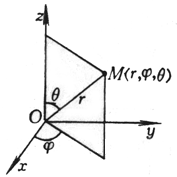r为矢径长(OM)，j 为经度，q 为纬度(或极距角) 这里 0 £ r < ¥ - ¥ < j < ¥ 0 £ q £ p [圆柱面坐标与直角坐标的互换][球面坐标与直角坐标的互换]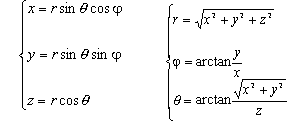[坐标轴的平移]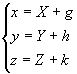式中x, y, z为旧坐标；X, Y, Z为新坐标；g, h, k为新坐标系原点O¢ 在旧坐标系内的坐标 [坐标轴的旋转]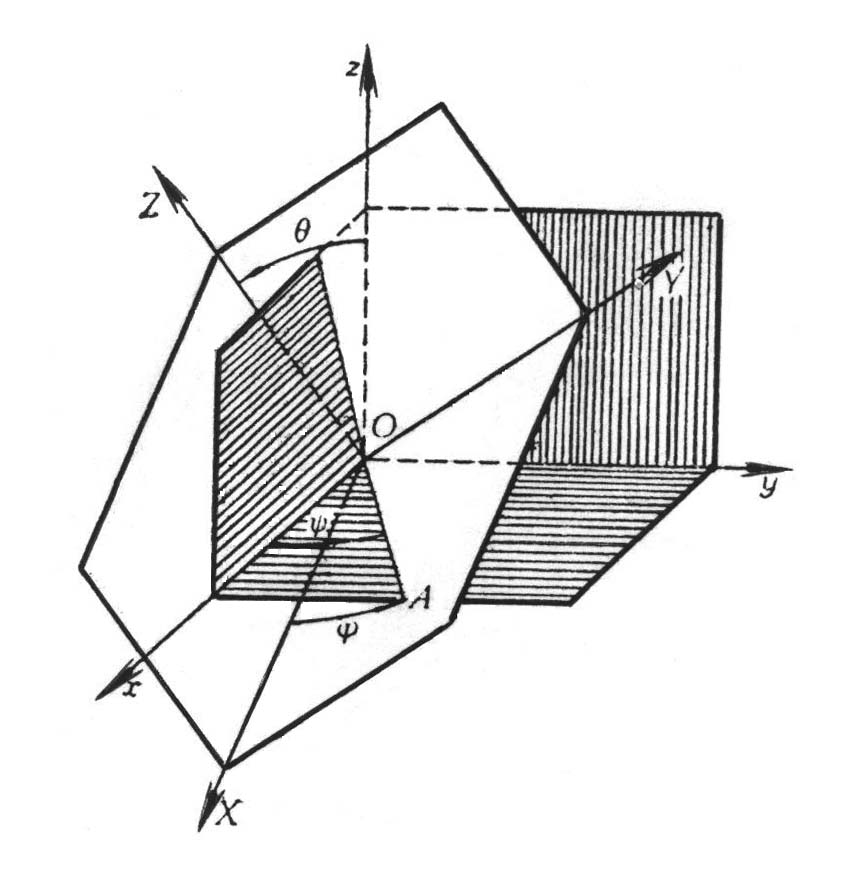按下表给出新坐标轴OX，OY，OZ的方向余弦时 新坐标轴 方向余弦 (见§ 4) OX OY OZ l1 m1 n1 l2 m2 n2 l3 m3 n3 则有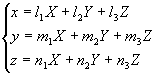[欧拉角] 新坐标轴的位置也可以用三个所谓欧拉角来确定(见上图)

1. 章动角q OZOz两轴正向夹角(0£ q < p ).
2. 进动角yOAOx的夹角(0£ y < 2p )，OAOXYOxy两平面的交线，面对Oz轴的正向，y 按逆时针方向从Ox轴开始计算.
3. 自转角jOAOX的夹角(0£ j < 2p )，面对OZ轴正向，j 按逆时针方向从OX轴开始计算
`若设`
`              c1=cosθ，	c2=cosy ，	c3=cosj `
`                     s1=sinθ，	s2=siny ，	s3=sinj `
` `
`则`
`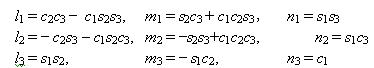`
```
变换行列式 Δ=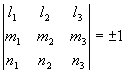```
` 当右手系变为右手系(或左手系变为左手系)时，Δ=1.当右手系变为左手系(或左手系变`
` 为右手系)时，Δ= -1 .`

`　`